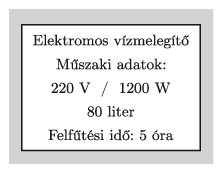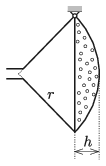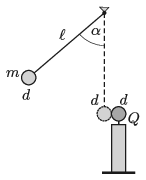Mathematical and Physical Journal
for High Schools
Issued by the MATFUND Foundation
 Already signed up? New to KöMaL?

# KöMaL Problems in Physics, October 2019

Show/hide problems of signs:## Problems with sign 'M'

Deadline expired on November 11, 2019.

M. 389. Attach a piece of thread to an egg and place the egg into a cylinder-shaped container. Pour water into the container such that it covers the egg, and then carefully pull out the egg from the water with the help of the thread.

Measure how the tension in the thread depends on the displacement of the egg. Determine the work done, while the egg was pulled out. Does this work depend on the cross section of the container?

(6 pont)

statistics## Problems with sign 'G'

Deadline expired on November 11, 2019.

G. 681. An artefact made of pure gold was found and dug out in good condition during an excavation at an archaeological site. The 2 litre cylinder-shaped artefact has thin uniform walls, and has no top base. The inner diameter and the inner height of the cylinder are equal.

When the empty cylinder is carefully placed into water such that its symmetry axis is vertical all the time, then it reaches an equilibrium position when the level of the water outside is at $\displaystyle \frac58$-th of the inner height of the cylinder. Determine the width of the wall of the artefact.

(3 pont)

solution (in Hungarian), statistics

G. 682. The data in the figure can be read on the label of an old boiler.$\displaystyle a)$ What is the efficiency of the boiler if during the warm-up time (5 hours) the boiler heats up water from a temperature of $\displaystyle 15\;{}^\circ$C to $\displaystyle 75\;{}^\circ$C?

$\displaystyle b)$ Today this boiler is used at a voltage of 230 V. To what time did the warm-up time of the boiler decreased?

(3 pont)

solution (in Hungarian), statistics

G. 683. We have two alike (red) resistors and also two alike (blue) resistors. In which connection will the equivalent resistance of the resistors be greater, if

$\displaystyle a)$ the two red resistors and the two blue resistors are connected in series, and then these are connected in parallel;

$\displaystyle b)$ the series connections of a red and a blue resistor are connected in parallel?

(4 pont)

solution (in Hungarian), statistics

G. 684. A plane – from cartographic purposes – flies at a constant speed for quite a long time above the equator, at some small height. The operators at the ground observe that the plane passes its starting position at every 48-th hour. How much time elapses between the sunset and the sunrise on the plane if the plane flies

$\displaystyle a)$ towards the east,

$\displaystyle b)$ towards the west?

(From time to time the plane is filled with fuel in the air.)

(4 pont)

solution (in Hungarian), statistics## Problems with sign 'P'

Deadline expired on November 11, 2019.

P. 5154. A boy is riding his bicycle in a cinema scene. When the boy rides his bicycle slowly, the wheels seem to rotate in a direction which is in accordance with the motion of the bicycle. When the boy speeds up, suddenly the wheels seem to turn in the opposite direction. When the speed of the bicycle is further increased, the apparent rotation of the wheels slows down gradually (but they still rotate in the wrong'' direction), and at some particular speed of $\displaystyle v$ the wheels seem to stop rotating. What is the value of $\displaystyle v$ if the perimeter of the wheels is 2.5 m, each wheel has 36 spikes, and the film has 24 frames in a second?

(4 pont)

solution (in Hungarian), statistics

P. 5155. A symmetrical letter E was bent from a thin piece of wire of length $\displaystyle (n+4)\ell$ such that each of its horizontal parts has a length of $\displaystyle \ell$ and its vertical part has a length of $\displaystyle n\ell$. Where is the centre of mass of the figure?

(4 pont)

solution (in Hungarian), statistics

P. 5156. The spherical sector shaped rose of a watering can, made of thin metal, is hung at one point on its circular rim by means of a hinge (see the figure). What is the ratio $\displaystyle h/r$, if in the equilibrium position of the rose its symmetry axis is horizontal? (The metal sheet has constant width and uniform density. The size of the tube through which the water enters the rose, as well as the total area of the small holes can be considered negligibly small.)(5 pont)

solution (in Hungarian), statistics

P. 5157. Which bullet, used in target shooting, is deflected more by the fictitious force due to the rotation of the Earth (called Coriolis force) a faster or a slower one?

(4 pont)

solution (in Hungarian), statistics

P. 5158. Using the data of tables determine the error by which a sample of saturated water vapour in a pressure-cooker at a temperature of $\displaystyle 120\;{}^\circ\rm C$ can be considered ideal with respect to density.

(3 pont)

solution (in Hungarian), statistics

P. 5159. An electric stove, which has three power levels, was made from two alike resistors. When it is used from the mains at the highest heat level it delivers a power of 1500 W. What is the resistance of the resistors? How long does it take to heat half a litre of water from $\displaystyle 20\;{}^\circ\rm C$ to $\displaystyle 80\;{}^\circ\rm C$ if it is used in its lowest heat level and its efficiency is 75%?

(3 pont)

solution (in Hungarian), statistics

P. 5160. The charge on a metal sphere of diameter $\displaystyle d=2$ cm, mounted on a fixed insulating stand, is $\displaystyle Q=8\cdot10^{-9}$ C. Another, but a neutral metal sphere of mass $\displaystyle m=1$ g and of the same size as the previously described one, is attached to a thin insulating piece of thread of length $\displaystyle \ell=1$ m as shown in the figure. The thread is displaced by an angle of $\displaystyle \alpha=60^\circ$, and then it is released. The two spheres collide head on, totally elastically. The energy of the electric field does not change in the course of the collision, there is no energy dissipation. How much higher above its initial position will the sphere on the thread go if air drag is also negligible?(5 pont)

solution (in Hungarian), statistics

P. 5161. A very long uncharged metal cylinder of base radius $\displaystyle R$ is placed into strong uniform magnetic field of magnetic induction $\displaystyle \boldsymbol B$. The axle through the symmetry axis of the cylinder is fixed parallel to the magnetic induction, and then the cylinder is started to rotate about by angular velocity $\displaystyle \omega$. What is the resulted surface charge density on the lateral surface of the cylinder?

(5 pont)

solution (in Hungarian), statistics

P. 5162. The faces of an equilateral-triangle-based right prism are plane mirrors. The prism executes uniform rotational motion of period $\displaystyle T$ about a vertical axis which goes through the centroid of the horizontal base of the prism. A horizontal beam of laser light aimed at the axis of rotation is incident on one of the faces. At the instant $\displaystyle t=0$ the beam is perpendicular to one of the mirrors. Determine and graph the angle between the incident and the reflected laser beam as a function of the elapsed time over the interval $\displaystyle 0\le t\le T$.

(4 pont)

solution (in Hungarian), statistics

P. 5163. The nuclide of a cobalt isotope of atomic mass number 60 emits an electron when it decays. What is the resulting nucleus?

(3 pont)

solution (in Hungarian), statistics